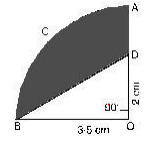# Class 10 Maths Assignments for Areas Related to Circles

In this page we have Class 10 Maths Assignments for Areas Related to Circles . Hope you like them and do not forget to like , social share and comment at the end of the page.

1) In figure, OACB is a quadrant of a circle with centre O and radius 3.5cm. If OD = 2cm, find the area of the2) From each of the two opposite corners of a square of side 8cm, a quadrant of a circle of radius 1.4cm is cut. Another circle of diameter 4.2cm is also cut from the centre as shown in figure. Find the area of the remaining (shaded) portion of the square. (Use π=22/7)
3) From a thin metallic piece, in the shape of a trapezium ABCD, in which AB//CD and <BCD = 90o, a quarter circle BEFC is removed. Given AB = BC = 3.5cm. and DE = 2cm, calculate the area of the remaining piece of the metal sheet.

4) The wheel of a motor cycle is of radius 35 cm. How many revolutions per minute must the wheel make so as to keep a speed of 66 km/h?
5) The short and long hands of a clock are 4cm and 6cm long respectively. Find the sum of distances travelled by their tip in 2 days. (Take π=22/7)
6) An arc of a circle is of length 5π cm and the sector it bounds has an area of 20π cm2. Find the radius of the circle.Go back to Class 10 Main Page using below links

### Practice Question

Question 1 What is $1 - \sqrt {3}$ ?
A) Non terminating repeating
B) Non terminating non repeating
C) Terminating
D) None of the above
Question 2 The volume of the largest right circular cone that can be cut out from a cube of edge 4.2 cm is?
A) 19.4 cm3
B) 12 cm3
C) 78.6 cm3
D) 58.2 cm3
Question 3 The sum of the first three terms of an AP is 33. If the product of the first and the third term exceeds the second term by 29, the AP is ?
A) 2 ,21,11
B) 1,10,19
C) -1 ,8,17
D) 2 ,11,20